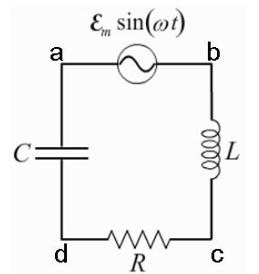# Problem: A circuit is constructed with an AC generator, a resistor, capacitor and inductor as shown. The generator voltage varies in time as E =Va - Vb = εmsinwt, where εm = 120 V and w = 783 radians: second. The values for the remaining circuit components are: R = 86 0, L = 75.7 mH, and C = 12.7MuF. The value for L is unkown. What is known is that the voltage across the generator leads the current in the circuit by φ = 45 degrees.What is t1, the first time after t = 0 when the voltage across the inductor is zero?

###### FREE Expert Solution

Time, t1 is expressed as:

$\overline{){{\mathbf{t}}}_{{\mathbf{1}}}{\mathbf{=}}\frac{\mathbf{\phi }}{\mathbf{\omega }}}$

Convert ω to degrees/sec

82% (361 ratings)###### Problem Details

A circuit is constructed with an AC generator, a resistor, capacitor and inductor as shown. The generator voltage varies in time as E =Va - Vb = εmsinwt, where εm = 120 V and w = 783 radians: second. The values for the remaining circuit components are: R = 86 0, L = 75.7 mH, and C = 12.7MuF. The value for L is unkown. What is known is that the voltage across the generator leads the current in the circuit by φ = 45 degrees.

What is t1, the first time after t = 0 when the voltage across the inductor is zero?